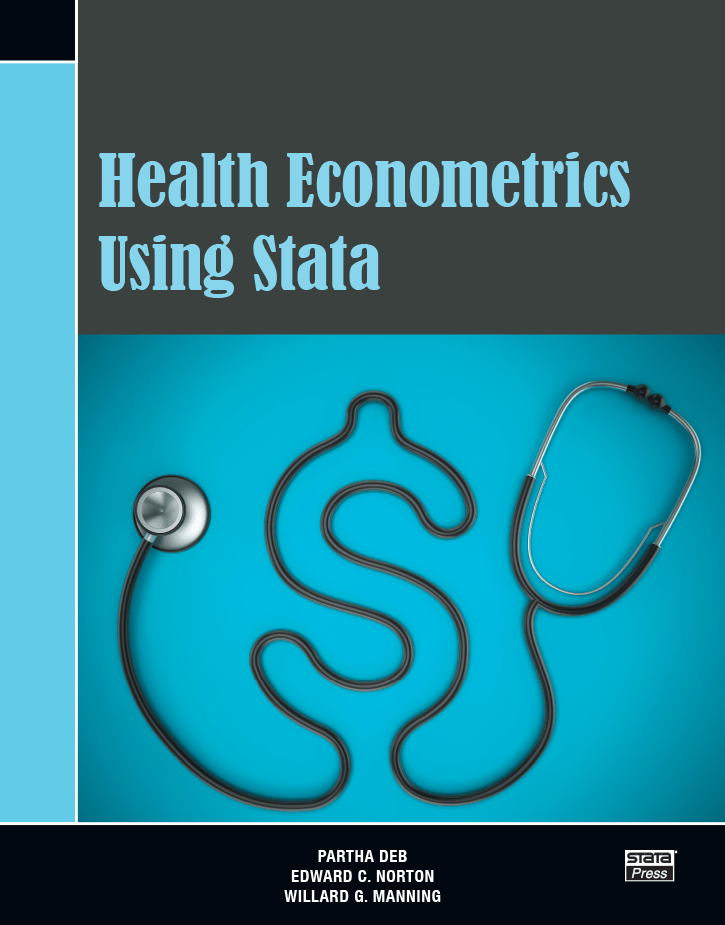# Health Econometrics Using Stata

Health Econometrics Using Stata by Partha Deb, Edward C. Norton, and Willard G. Manning provides an excellent overview of the methods used to analyze data on healthcare expenditure and use. Aimed at researchers, graduate students, and practitioners, this book introduces readers to widely used methods, shows them how to perform these methods in Stata, and illustrates how to interpret the results. Each method is discussed in the context of an example using an extract from the Medical Expenditure Panel Survey.

After the overview chapters, the book provides excellent introductions to a series of topics aimed specifically at those analyzing healthcare expenditure and use data. The basic topics of linear regression, the generalized linear model, and log and Box-Cox models are covered with a tight focus on the problems presented by these data. Using this foundation, the authors cover the more advanced topics of models for continuous outcome with mass points, count models, and models for heterogeneous effects. Finally, they discuss endogeneity and how to address inference questions using data from complex surveys.

The authors use their formidable experience to guide readers toward useful methods and away from less recommended ones. Their discussion of “health econometric myths” and the chapter presenting a framework for approaching health econometric estimation problems are especially useful for this aspect.

List of tables

List of figures

Preface

Notation and typography

1. INTRODUCTION

Outline

Themes

Health econometric myths

Stata friendly

A useful way forward

2. FRAMEWORK

Introduction

Potential outcomes and treatment effects

Estimating ATEs

A laboratory experiment

Randomization

Regression estimates of treatment effects

Linear regression

Nonlinear regression

Incremental and marginal effects

Model selection

In-sample model selection

Cross-validation

Other issues

3. MEPS DATA

Introduction

Overview of all variables

Expenditure and use variables

Explanatory variables

Sample dataset

Stata resources

4. THE LINEAR REGRESSION MODEL: SPECIFICATION AND CHECKS

Introduction

The linear regression model

Marginal, incremental, and treatment effects

Marginal and incremental effects

Graphical representation of marginal and incremental effects

Treatment effects

Consequences of misspecification

Example: An exponential specification

Visual checks

Artificial-data example of visual checks

MEPS example of visual checks

Statistical tests

Ramsey’s RESET test

Modified Hosmer–Lemeshow test

Examples

Model selection using AIC and BIC

Stata resources

5. GENERALIZED LINEAR MODELS

Introduction

GLM framework

GLM assumptions

Parameter estimation

GLM examples

GLM predictions

GLM example with interaction term

Marginal and incremental effects

Example of marginal and incremental effects

Choice of link function and distribution family

AIC and BIC

Modified Park test for the distribution family

Extended GLM

Conclusions

Stata resources

6. LOG AND BOX – COX MODELS

Introduction

Log models

Log model estimation and interpretation

Retransformation from ln(y) to raw scale

Error retransformation and model predictions

Marginal and incremental effects

Comparison of log models to GLM

Box–Cox models

Box–Cox example

Stata resources

7. MODELS FOR CONTINUOUS OUTCOMES WITH MASS AT ZERO

Introduction

Two-part models

Expected values and marginal and incremental effects

Generalized tobit

Full-information maximum likelihood and limited-information maximum likelihood

Comparison of two-part and generalized tobit models

Examples that show similarity of marginal effects

Interpretation and marginal effects

Two-part model example

Two-part model marginal effects

Two-part model marginal effects example

Generalized tobit interpretation

Generalized tobit example

Single-index models that accommodate zeros

The tobit model

Why tobit is used sparingly

One-part models

Statistical tests

Stata resources

8. COUNT MODELS

Introduction

Poisson regression

Poisson MLE

Robustness of the Poisson regression

Interpretation

Is Poisson too restrictive?

Negative binomial models

Examples of negative binomial models

Hurdle and zero-inflated count models

Hurdle count models

Zero-inflated models

Truncation and censoring

Truncation

Censoring

Model comparisons

Model selection

Cross-validation

Conclusion

Stata resources

9. MODELS FOR HETEROGENEOUS EFFECTS

Introduction

Quantile regression

MEPS examples

Extensions

Finite mixture models

MEPS example of healthcare expenditures

MEPS example of healthcare use

Nonparametric regression

MEPS examples

Conditional density estimator

Stata resources

10. ENDOGENEITY

Introduction

Endogeneity in linear models

OLS is inconsistent

2SLS

Specification tests

2SRI

Modeling endogeneity with ERM

Endogeneity with a binary endogenous variable

GMM

Stata resources

11. DESIGN EFFECTS

Introduction

Features of sampling designs

Weights

Clusters and stratification

Weights and clustering in natural experiments

Methods for point estimation and inference

Point estimation

Standard errors

Empirical examples

Survey design setup

Weighted sample means

Weighted least-squares regression

Weighted Poisson count model

Conclusion

Stata resources

References

Author index

Subject indexAuthor: Partha Deb, Edward C. Norton, Willard G. Manning
ISBN-13: 978-1-59718-228-7